# reflectorGrid

Create grid reflector-backed antenna

Since R2020b

## Description

The `reflectorGrid` object creates a grid reflector-backed antenna. The grid reflector uses a grid of parallel wires or bars oriented in one direction. Grid reflectors can be used as high-gain antennas in point-to-point communications.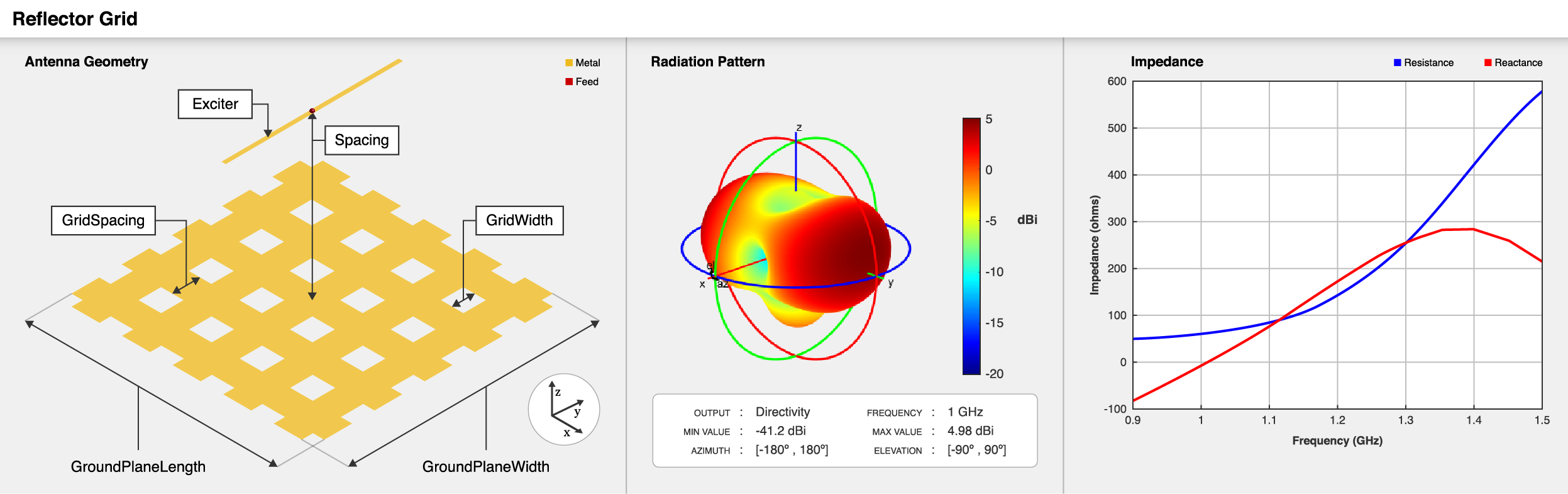## Creation

### Syntax

``ant = reflectorGrid``
``ant = reflectorGrid(Name=Value)``

### Description

example

````ant = reflectorGrid` creates a grid reflector-backed antenna. The default antenna object has an exciter as a dipole with the feed point located at the origin on the X-Y plane, and the antenna dimensions are chosen for an operating frequency of 1 GHz.```

example

````ant = reflectorGrid(Name=Value)` creates a grid reflector antenna, with additional Properties specified by one or more name–value arguments. `Name` is the property name and `Value` is the corresponding value. You can specify several name-value arguments in any order as `Name1`= `Value1`, `...`, `NameN`=`ValueN`. Properties not specified retain their default values.For example, `reflectorGrid(GroundPlaneWidth=0.6)` creates a grid reflector with a width of 0.6 meters. You can specify multiple name-value pairs.```

## Properties

expand all

Exciter antenna or array type, specified as a single-element antenna object, an array object, or an empty array. Except for reflector and cavity antenna elements, you can use any Antenna Toolbox™ antenna or array element as an exciter. To create the cavity backing structure without the exciter, specify this property as an empty array.

Example: `horn`

Example: `linearArray(Element=patchMicrostrip)`

Example: `[]`

Distance between reflector and exciter, specified as a positive scalar in meters.

Example: `0.259`

Data Types: `double`

Reflector length along the X-axis, specified as a positive scalar in meters.

Example: `0.6`

Data Types: `double`

Reflector width along the Y-axis, specified as a positive scalar in meters.

Example: `0.6`

Data Types: `double`

Type of the grid used in the reflector, specified as either one of the following:

• `"H"` — grids are arranged horizontally in the reflector.

• `"V"` — grids are arranged vertically in the reflector.

• `"HV"` or `"VH"` — grids are arranged both horizontally and vertically in the reflector.

Example: `"H"`

Data Types: `char`

Distance between the two grid cells, specified as a positive scalar in meters.

Example: `0.018`

Data Types: `double`

Width of each grid cell, specified as a positive scalar in meters.

Example: `0.3`

Data Types: `double`

Type of the metal used as a conductor, specified as a metal material object. You can choose any metal from the `MetalCatalog` or specify a metal of your choice. For more information, see `metal`. For more information on metal conductor meshing, see Meshing.

Example: `metal("Copper")`

Tilt angle of the antenna, specified as a scalar or vector with each element unit in degrees. For more information, see Rotate Antennas and Arrays.

Example: `Tilt=90`

Example: `Tilt=[90 90]`,`TiltAxis=[0 1 0;0 1 1]` tilts the antenna at 90 degrees about the two axes defined by the vectors.

Note

The `wireStack` antenna object only accepts the dot method to change its properties.

Data Types: `double`

Tilt axis of the antenna, specified as:

• Three-element vector of Cartesian coordinates in meters. In this case, each coordinate in the vector starts at the origin and lies along the specified points on the X-, Y-, and Z-axes.

• Two points in space, each specified as three-element vectors of Cartesian coordinates. In this case, the antenna rotates around the line joining the two points in space.

• A string input describing simple rotations around one of the principal axes, 'X', 'Y', or 'Z'.

Example: `TiltAxis=[0 1 0]`

Example: `TiltAxis=[0 0 0;0 1 0]`

Example: `TiltAxis = 'Z'`

Data Types: `double`

Lumped elements added to the antenna feed, specified as a `lumpedElement` object. You can add a load anywhere on the surface of the antenna. By default, the load is at the feed. For more information, see `lumpedElement`.

Example: `Load=lumpedelements`, where `lumpedelements` is the load added to the antenna feed.

Example: `lumpedElement(Impedance=75)`

## Object Functions

 `show` Display antenna, array structures or shapes `impedance` Input impedance of antenna; scan impedance of array `sparameters` Calculate S-parameter for antenna and antenna array objects `returnLoss` Return loss of antenna; scan return loss of array `vswr` Voltage standing wave ratio of antenna `pattern` Radiation pattern and phase of antenna or array; Embedded pattern of antenna element in array `patternAzimuth` Azimuth pattern of antenna or array `patternElevation` Elevation pattern of antenna or array `axialRatio` Axial ratio of antenna `beamwidth` Beamwidth of antenna `current` Current distribution on antenna or array surface `charge` Charge distribution on antenna or array surface `efficiency` Radiation efficiency of antenna `EHfields` Electric and magnetic fields of antennas; Embedded electric and magnetic fields of antenna element in arrays `mesh` Mesh properties of metal, dielectric antenna, or array structure `optimize` Optimize antenna or array using SADEA optimizer `design` Design prototype antenna or arrays for resonance around specified frequency `rcs` Calculate and plot radar cross section (RCS) of platform, antenna, or array `numGridsToSpacing` Calculate grid spacing in grid for `reflectorGrid` object

## Examples

collapse all

Create and view a grid reflector-backed antenna object with default properties.

```ant = reflectorGrid; show(ant)```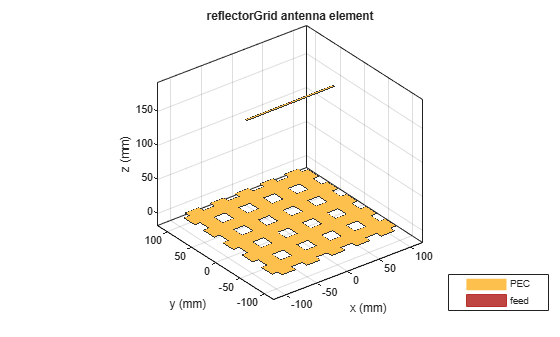Plot the radiation pattern of the antenna at 1 GHz.

`pattern(ant,1e9)`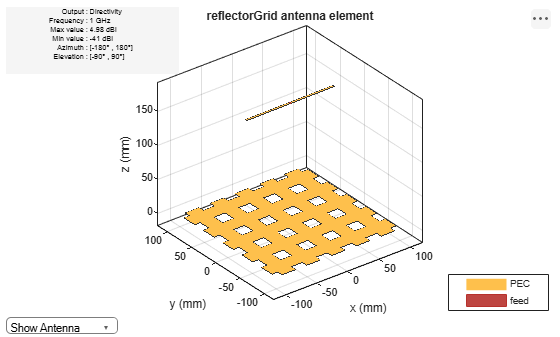Create and view a grid reflector-backed biquad antenna with an arm length of 0.01 meters.

```b = biquad(ArmLength=0.01); h = reflectorGrid(Exciter=b); show(h)```Plot the radiation pattern of the antenna at 0.6 GHz.

`pattern(h,0.6e9)`Create and view grid reflector-baked dipole blade antenna.

```d = dipoleBlade(Length=0.1,TaperLength=0.05,FeedGap=0.002); h = reflectorGrid(Exciter=d); show(h)```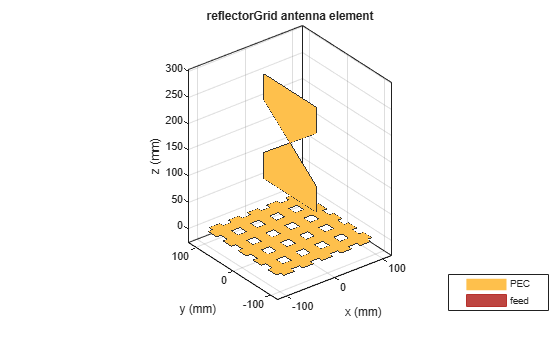Change the grid type from "`HV"` to "`H"`.

`h.GridType = "H";`

View the antenna with grid type "`H"`.

`show(h)`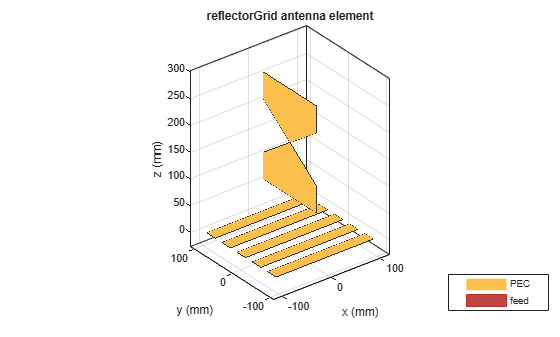Plot the radiation pattern at 1 GHz.

`pattern(h,1e9)`Create a rectangular array of cylindrical dipole antennas.

```d = dipoleCylindrical(Length=0.2,Radius=0.005); arr = rectangularArray(Element=d,Size=[4 4],RowSpacing=0.029,ColumnSpacing=0.029);```

Create a grid reflector-backed rectangular array.

`ant = reflectorGrid(Exciter=arr,Spacing=0.2)`
```ant = reflectorGrid with properties: Exciter: [1x1 rectangularArray] Spacing: 0.2000 GroundPlaneLength: 0.2000 GroundPlaneWidth: 0.2000 GridType: 'HV' GridSpacing: 0.0180 GridWidth: 0.0220 Conductor: [1x1 metal] Tilt: 0 TiltAxis: [1 0 0] Load: [1x1 lumpedElement] ```
`show(ant)`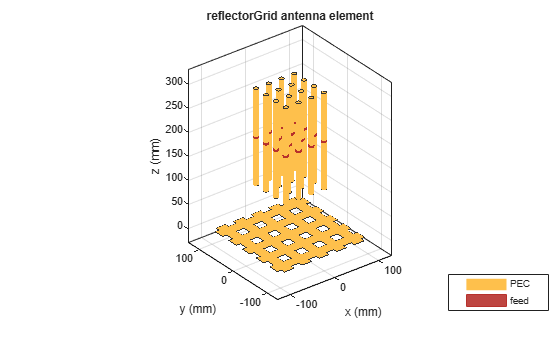Create and visualize a grid reflector-backed antipodal Vivaldi antenna.

`ant = reflectorGrid(Exciter=vivaldiAntipodal)`
```ant = reflectorGrid with properties: Exciter: [1x1 vivaldiAntipodal] Spacing: 0.1750 GroundPlaneLength: 0.2000 GroundPlaneWidth: 0.2000 GridType: 'HV' GridSpacing: 0.0180 GridWidth: 0.0220 Conductor: [1x1 metal] Tilt: 0 TiltAxis: [1 0 0] Load: [1x1 lumpedElement] ```
`show(ant)`This example shows how to create and analyze a planar reflector grid structure without any exciter element, using `planeWaveExcitation`.

Create default reflector grid antenna

Create a reflector grid antenna operating at 1 GHz using the `design` function and the `reflectorGrid` element from the antenna catalog, and view it.

```f=1e9; ant=design(reflectorGrid,f); figure; show(ant)```Derive backing structure

Derive the backing structure from this reflector grid antenna by assigning empty value to the `'Exciter'` property and view it.

`ant.Exciter=[]`
```ant = reflectorGrid with properties: Exciter: [] Spacing: 0.1749 GroundPlaneLength: 0.2000 GroundPlaneWidth: 0.2000 GridType: 'HV' GridSpacing: 0.0180 GridWidth: 0.0220 Conductor: [1x1 metal] Tilt: 0 TiltAxis: [1 0 0] Load: [1x1 lumpedElement] ```
```figure; show(ant)```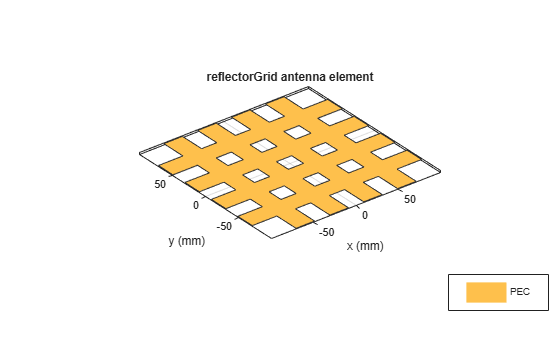Mesh reflector grid

Mesh the grid structure with a maximum edge length of 10 mm.

```figure; mesh(ant,MaxEdgeLength=10e-3)```Plot surface current distribution

Use planewave excitation to excite the grid structure and plot the surface current distribution at 1 GHz.

`pw=planeWaveExcitation(Element=ant,Direction=[0 0 -1],Polarization=[1 0 0])`
```pw = planeWaveExcitation with properties: Element: [1x1 reflectorGrid] Direction: [0 0 -1] Polarization: [1 0 0] SolverType: 'MoM' ```
```figure; current(pw,f,Scale="log10")```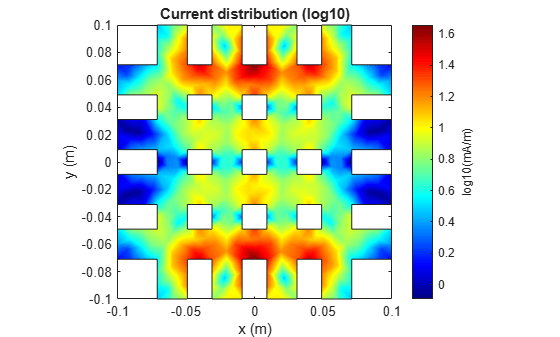Balanis, Constantine A. Antenna Theory: Analysis and Design. 3rd ed. Hoboken, NJ: John Wiley, 2005.